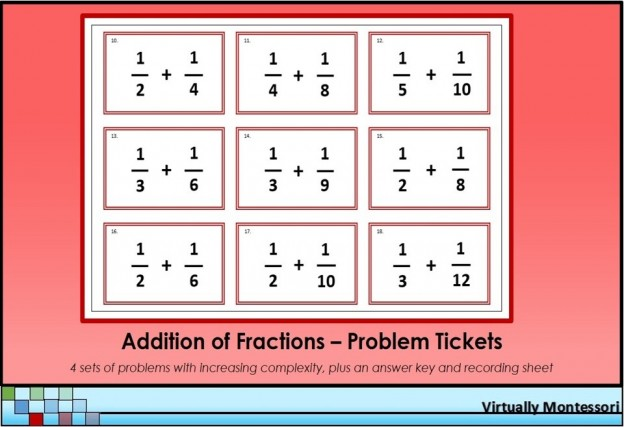# Addition of Fractions Problem Tickets

These fraction problems are intended to be follow-up practice after a lesson. Each group of problems adds a new layer of complexity. The red border represents the addition operation in Montessori math lessons and materials.

After giving a lesson, chosen tickets are handed by the teacher to the student as his follow-up practice. The student completes the work on his own, records on the recording sheet or in a math journal, then checks his work using the answer key. Each problem ticket has a number on it that corresponds with the answer key. The tickets can also be cut up and placed in a basket for use as a math activity or center.

The following items are included in this material:
— Addition of fractions problem tickets
—– Problems #1-9: Adding simple fractions with the same denominator (these can be used with the Montessori metal fraction insets or fraction circles)
—– Problems #10-18: Adding fractions with different denominators: one addend needs to be changed
—– Problems #19-27: Adding fractions with different denominators: both addends need to be changed
—– Problems #28-36: Adding fractions with mixed numbers: both addends need to be changed